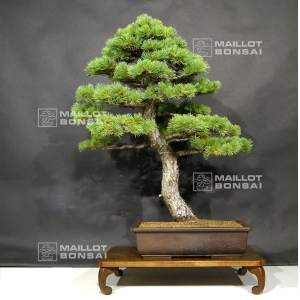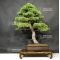##### The Japanese Bonsai specialist
Direct order Contact Help / Services Newsletter# Pinus pentaphylla ref:23070181

Pinus pentaphyllaref. : 10000

1.340,00

voluminous/heavy item extra shipping of 12,00

Available quantity : 1Order

###### Description

Height of the tree without its pot : 780 mm. Width of the branches : 720 mm. Japanese brown non-enamelled pottery of : 450 x 320 x 110 mm. Moyogi style with a sinuous trunk. Pine with a regular silhouette, a large perfectly-conical trunk without any very strong wire marks or cutting wounds. The trunk’s diameter amounts 80 mm. Good-looking blue-toned needles. Pinus species coming from seeding selected in nature (yamadori) more than 30 years ago. This tree is composed of a formed bark because it is aged of more than 40-45 years old. Look the beauty of the trunk, its movement, its conical aspect. Coming from master Obitsu’s nursery. Currently ligatured with aluminium wire, you will require to remove the wire in winter 2018-2019. Repot this tree in an oval pottery with less depth will enable to better highlight this good-looking tree.

Photographed in july 2018. Shelf non-included.

#trunk 4.5 #tree 4.4 #with 4.4 #pinus 3.5 #wire 3.4 #this 3.4 #pentaphylla 3.1 #coniferous 3 #without 2.7 #looking 2.7

Formule
(( ROUND((CHAR_LENGTH(b.article_nom)-CHAR_LENGTH(REPLACE(b.article_nom, 'trunk', '')))/LENGTH('trunk')) + ROUND((CHAR_LENGTH(b.article_description)-CHAR_LENGTH(REPLACE(b.article_description, 'trunk', '')))/LENGTH('trunk')) ) * 4.5) + (( ROUND((CHAR_LENGTH(b.article_nom)-CHAR_LENGTH(REPLACE(b.article_nom, 'with', '')))/LENGTH('with')) + ROUND((CHAR_LENGTH(b.article_description)-CHAR_LENGTH(REPLACE(b.article_description, 'with', '')))/LENGTH('with')) ) * 4.4) + (( ROUND((CHAR_LENGTH(b.article_nom)-CHAR_LENGTH(REPLACE(b.article_nom, 'tree', '')))/LENGTH('tree')) + ROUND((CHAR_LENGTH(b.article_description)-CHAR_LENGTH(REPLACE(b.article_description, 'tree', '')))/LENGTH('tree')) ) * 4.4) + (( ROUND((CHAR_LENGTH(b.article_nom)-CHAR_LENGTH(REPLACE(b.article_nom, 'pinus', '')))/LENGTH('pinus')) + ROUND((CHAR_LENGTH(b.article_description)-CHAR_LENGTH(REPLACE(b.article_description, 'pinus', '')))/LENGTH('pinus')) ) * 3.5) + (( ROUND((CHAR_LENGTH(b.article_nom)-CHAR_LENGTH(REPLACE(b.article_nom, 'this', '')))/LENGTH('this')) + ROUND((CHAR_LENGTH(b.article_description)-CHAR_LENGTH(REPLACE(b.article_description, 'this', '')))/LENGTH('this')) ) * 3.4) + (( ROUND((CHAR_LENGTH(b.article_nom)-CHAR_LENGTH(REPLACE(b.article_nom, 'wire', '')))/LENGTH('wire')) + ROUND((CHAR_LENGTH(b.article_description)-CHAR_LENGTH(REPLACE(b.article_description, 'wire', '')))/LENGTH('wire')) ) * 3.4) + (( ROUND((CHAR_LENGTH(b.article_nom)-CHAR_LENGTH(REPLACE(b.article_nom, 'pentaphylla', '')))/LENGTH('pentaphylla')) + ROUND((CHAR_LENGTH(b.article_description)-CHAR_LENGTH(REPLACE(b.article_description, 'pentaphylla', '')))/LENGTH('pentaphylla')) ) * 3.1) + (( ROUND((CHAR_LENGTH(b.article_nom)-CHAR_LENGTH(REPLACE(b.article_nom, 'conical', '')))/LENGTH('conical')) + ROUND((CHAR_LENGTH(b.article_description)-CHAR_LENGTH(REPLACE(b.article_description, 'conical', '')))/LENGTH('conical')) ) * 2.7) + (( ROUND((CHAR_LENGTH(b.article_nom)-CHAR_LENGTH(REPLACE(b.article_nom, 'looking', '')))/LENGTH('looking')) + ROUND((CHAR_LENGTH(b.article_description)-CHAR_LENGTH(REPLACE(b.article_description, 'looking', '')))/LENGTH('looking')) ) * 2.7) + (( ROUND((CHAR_LENGTH(b.article_nom)-CHAR_LENGTH(REPLACE(b.article_nom, 'pottery', '')))/LENGTH('pottery')) + ROUND((CHAR_LENGTH(b.article_description)-CHAR_LENGTH(REPLACE(b.article_description, 'pottery', '')))/LENGTH('pottery')) ) * 2.7)

## Secure payment## Delivery

Our logistic partners :